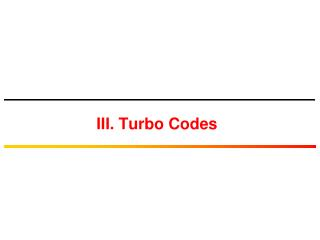DownloadDownload PresentationIII. Turbo Codes

# III. Turbo Codes

Download Presentation## III. Turbo Codes

- - - - - - - - - - - - - - - - - - - - - - - - - - - E N D - - - - - - - - - - - - - - - - - - - - - - - - - - -
##### Presentation Transcript

1. III. Turbo Codes

2. Turbo Decoding • Turbo decoders rely on probabilistic decoding of component RSC decoders • Iteratively exchanging soft-output information between decoder 1 and decoder 2 before making a final deciding on the transmitted bits • As the number of iteration grows, the decoding performance improves

3. Turbo Coded System Model u = [u1, …, uk, …,uN]: vector of N information bits xs = [x1s, …, xks, …,xNs] : vector of N systematic bits after turbo-coding of u x1p = [x11p, …, xk1p, …,xN1p] : vector of N parity bits from first encoder after turbo-coding of u x2p = [x12p, …, xk2p, …,xN2p] : vector of N parity bits from second encoder after turbo-coding of u x= [x1s, x11p, x12p, …, xNs, xN1p, xN2p] : vector of length 3N of turbo-coded bits for u y= [y1s, y11p, y12p, …, yNs, yN1p, yN2p] : vector of 3N received symbols corresponding to turbo-coded bits of u ys = [y1s, …, yks, …,yNs] : vector of N received symbols corresponding to systematic bits in xs y1p = [y11p, …, yk1p, …,yN1p] : vector of N received symbols corresponding to first encoder parity bits in x1p y2p = [y12p, …, yk2p, …,yN2p] : vector of N received symbols corresponding to second encoder parity bits in x2p u*= [u1*, …, uk*, …,uN*] : vector of N turbo decoder decision bits corresponding to u ys xs u=[u1, …, uk, …,uN] Turbo Decoding y1p x1p RSC Encoder 1 y x u* MUX DEMUX Channel N-bit Interleaver π y2p x2p RSC Encoder 2 Pr[uk|y] is known as the a posteriori probability of kth information bit

4. Log-Likelihood Ratio (LLR) The LLR of an information bit uk is given by: Given that Pr[uk=+1]+Pr[uk=-1]=1

5. Maximum A Posteriori Algorithm ys xs u=[u1, …, uk, …,uN] Turbo Decoding y1p x1p RSC Encoder 1 y x u* MUX DEMUX Channel N-bit Interleaver π y2p x2p RSC Encoder 2

6. Some Definitions & Conventions ys xs u=[u1, …, uk, …,uN] Turbo Decoding y1p x1p RSC Encoder 1 y x u* MUX DEMUX Channel N-bit Interleaver π y2p x2p RSC Encoder 2 uk ykp Sk Sk-1 s0 s0 s1 s1 s2 s2 uk=-1 uk=+1 s3 s3

7. Derivation of LLR uk ykp Sk Sk-1 Define S(i) as the set of pairs of states (s’,s) such that the transition from Sk-1=s’ to Sk=s is caused by the input uk=i, i=0,1, i.e., S(0) ={(s0 ,s0), (s1 ,s3), (s2 ,s1), (s3 ,s2)} S(1) ={(s0 ,s1), (s1 ,s2), (s2 ,s0), (s3 ,s3)} s0 s0 s1 s1 s2 s2 uk=-1 uk=+1 s3 s3 Remember Bayes’ Rule

8. Derivation of LLR Define

9. Derivation of LLR depends only on Sk and is independent on Sk-1, yk and Sk , yk depends only on Sk-1 and is independent on

10. Derivation of LLR

11. Derivation of LLR S0 Sk-1 Sk Sk+1 SN s0 s1 s2 s3 y= y1 yk-1 yk yk+1 yN

12. Computation of αk(s) Example

13. Computation of αk(s) Forward Recursive Equation Given the values of γk(s’,s) for all index k, the probability αk(s) can be forward recursively computed. The initial condition α0(s) depends on the initial state of the convolutional encoder The encoder usually starts at state 0

14. Computation of βk(s) Backward Recursive Equation Given the values of γk(s’,s) for all index k, the probability βk(s) can be backward recursively computed. The initial condition βN(s) depends on the final state of the trellis First encoder usually finishes at state s0 Second encoder usually has open trellis

15. Computation of γk(s’,s) Note that

16. Computation of γk(s’,s)